Function Repository Resource:

# TrigApproximateList

Generate a trig series that approximates a list of data

Contributed by: Jon McLoone
 ResourceFunction["TrigApproximateList"][data,var] generates a trig series in var which approximately matches equally spaced data. ResourceFunction["TrigApproximateList"][data,var,n] generates a trig series of n terms in var which approximately matches equally spaced data. ResourceFunction["TrigApproximateList"][{{x1,y1},{x2,y2},…},var,n] generates two trig series of n terms in var which, as a parametric function, approximately matches each column of data.

## Details and Options

ResourceFunction["TrigApproximateList"] uses the discrete Fourier transform of data to quickly establish the magnitude, frequency and phase of the dominant frequencies in a list of data and constructs a trigonometric series using these values.
ResourceFunction["TrigApproximateList"][data,var,n] calculates the low frequency half the discrete Fourier transform of data, selects all terms not matching the "TermSelectionFunction" and then takes up to n components that are maximal by "TermOrderingFunction". For each Fourier component remaining, an appropriate expression of the form aCos[bvar+c] is constructed according to the Fourier component.
Options accepted by ResourceFunction["TrigApproximateList"] include:
 DataRange Automatic the range of actual coordinates the data should be assumed to occupy "TermOrderingFunction" Abs the method for sorting the most significant Fourier components "TermSelectionFunction" True& a test for selecting Fourier components to use

## Examples

### Basic Examples (2)

Create an approximate formula for a sequence of values based on the discrete Fourier transform of the data:

 In:=Out=In:=Out=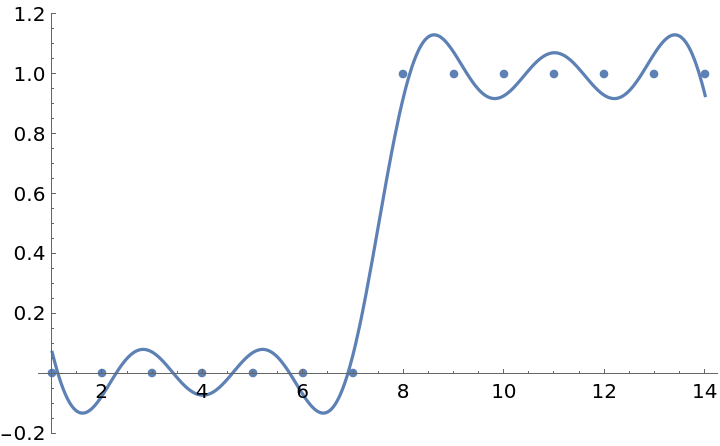A simpler approximation can be created by using only the three most dominant values from the discrete Fourier transform:

 In:=Out=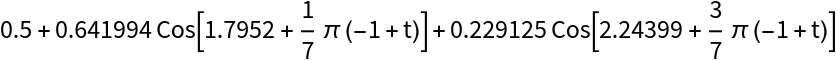In:=Out=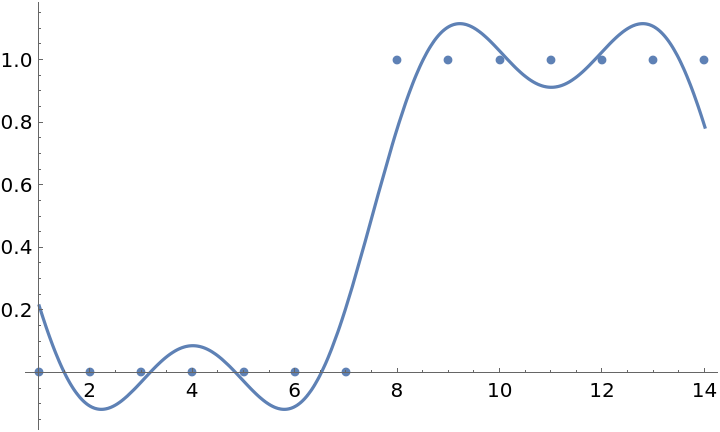### Scope (2)

For unevenly-spaced data or a path of points in 2D space, TrigApproximateList generates a pair of expressions using a common variable:

 In:=Out=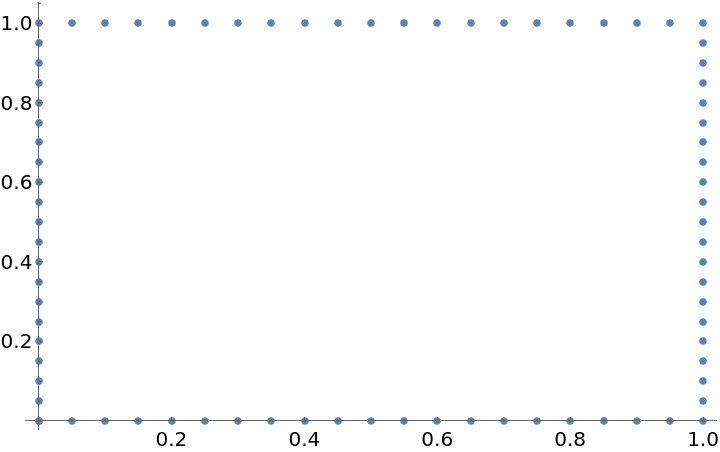In:=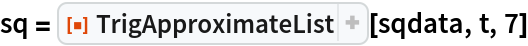Out=The output is appropriate for use within ParametricPlot to generate this approximation to a square:

 In:=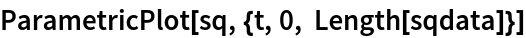Out=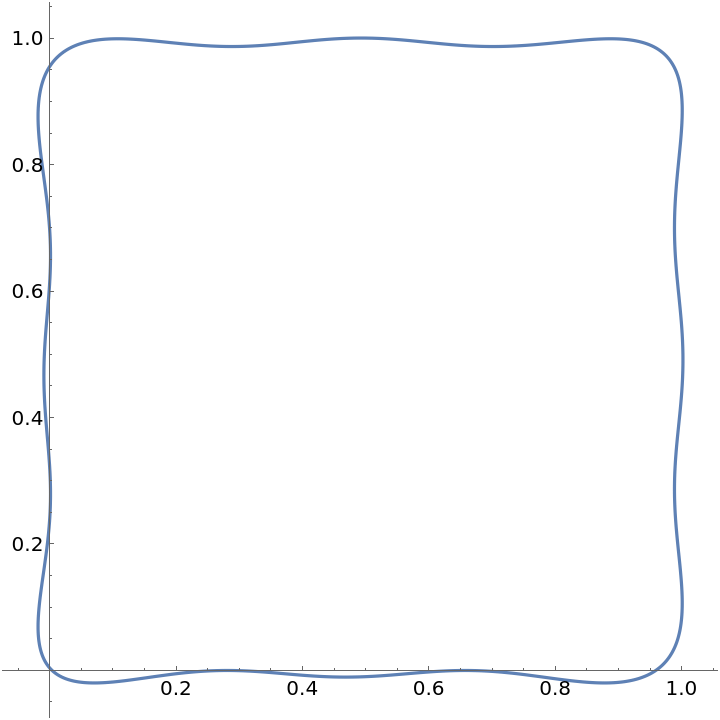More terms produce more accurate approximations:

 In:=Out=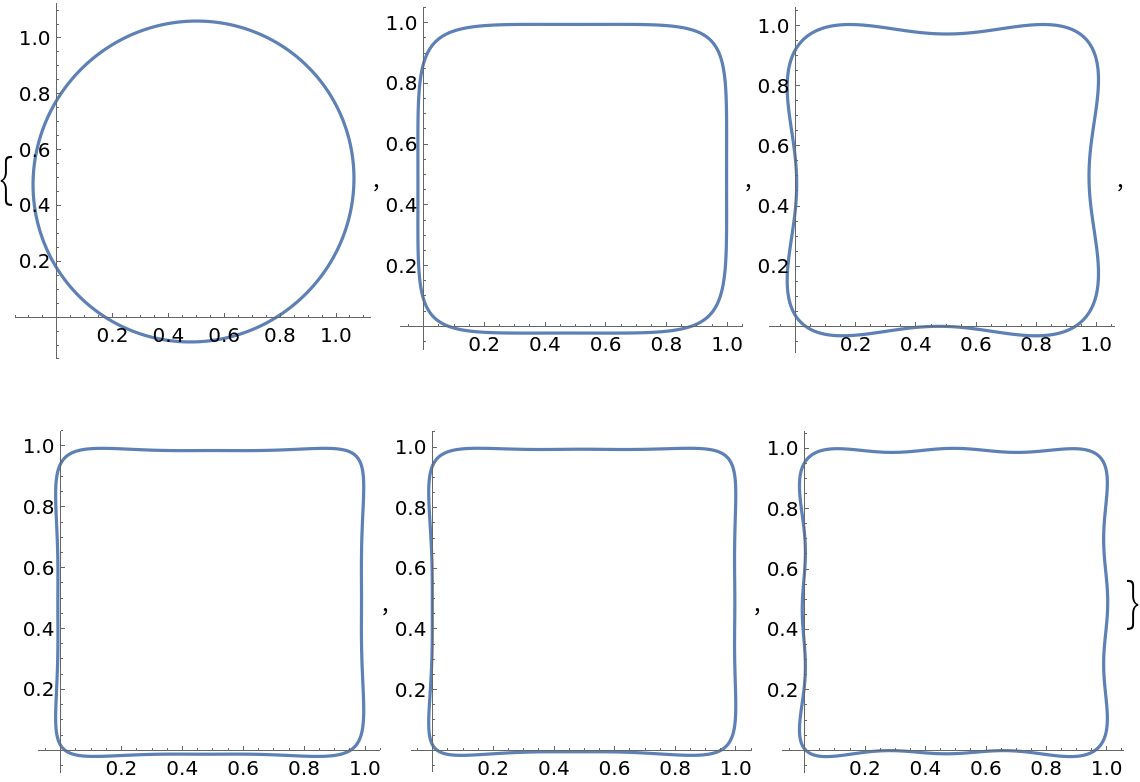When the number of terms is not specified, TrigApproximateList uses Length[data]/2 terms:

 In:=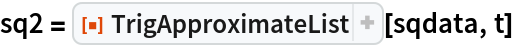Out=This produces the most accurate approximation possible while still preventing high-frequency over-fitting:

 In:=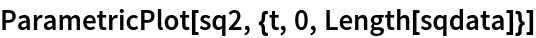Out=### Options (3)

The data range is assumed to be from 1 to Length[data]. To change this, use DataRange:

 In:=Out=In:=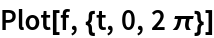Out=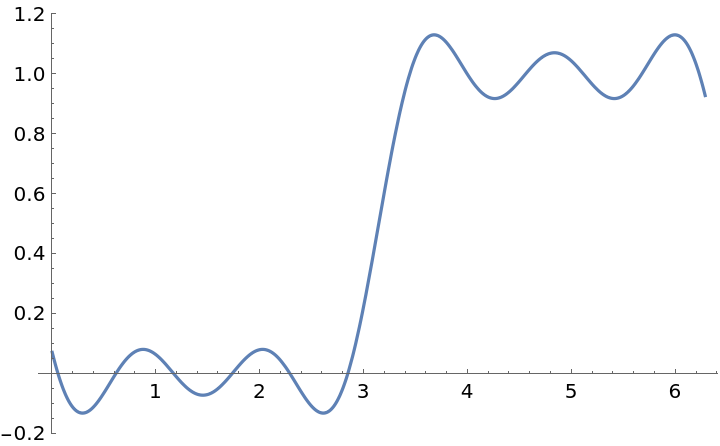Instead of specifying the number of terms to return, you can specify a criteria for deciding when a Fourier term is significant enough to use:

 In:=Out=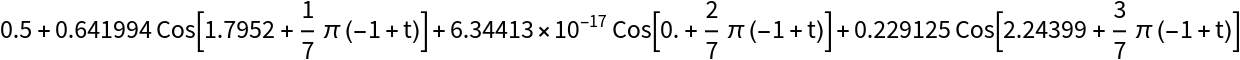When a fixed number of terms is requested, you can specify which terms are more important with "TermOrderingFunction". Here terms with the largest real component are used first:

 In:=Out=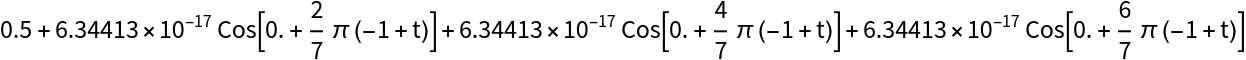### Neat Examples (1)

Create a smooth parametric outline from an image:

 In:=Out=In:=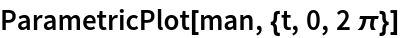Out=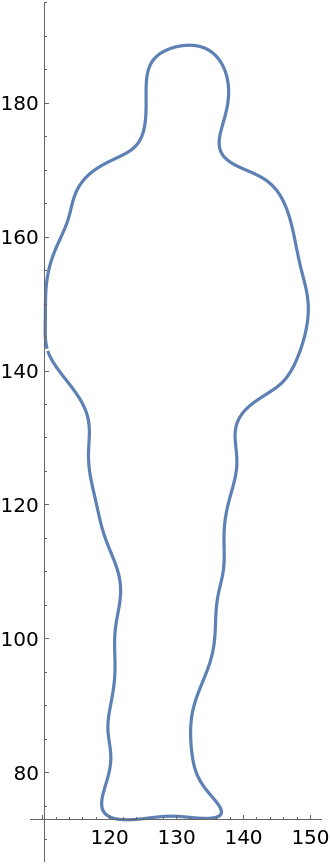Jon McLoone

## Version History

• 1.0.0 – 20 January 2022